Math resources Geometry Surface area

Surface area of a prism

# Surface area of a prism

Here you will learn about the surface area of a prism, including what it is and how to calculate it.

Students will first learn about the surface area of a prism as part of geometry in 6 th grade.

## What is the surface area of a prism?

The surface area of a prism is the total area of all of the faces of the prism. Prisms are 3D shapes made up of flat faces, including polygonal bases and rectangle faces.

One type of prism is a rectangular prism.

A rectangular prism is made up of 6 rectangular faces, including 2 rectangular bases and 4 rectangular lateral faces.

For example,

Here is a rectangular prism and its net.

It has 3 pairs of congruent faces, since the opposite faces are the same.

To calculate the surface area of the rectangular prism, calculate the area of each face and then add them together.

FaceArea

A=5 \times 8=40 \, cm^2

A=3 \times 8=24 \, cm^2

A=5 \times 3=15 \, cm^2

The surface area of the prism is the sum of the areas. Add each area twice, since each rectangle appears twice in the prism:

40+40+24+24+15+15=158 \mathrm{~cm}^2

The surface area of the rectangular prism is equal to 158 \mathrm{~cm}^2 .

Note: Surface area is measured in square units. For example, \mathrm{mm}^2, \mathrm{~cm}^2, \mathrm{~m}^2 , etc.

Another type of prism is a triangular prism.

A triangular prism is made up of 5 faces, including triangular bases and 3 rectangular lateral faces.

For example,

Here is a triangular prism and its net.

The base of this triangular prism is an isosceles triangle – two of the side lengths are equal.

Remember that the edges in a prism are always equal, so if you were to fold up the net, the 6.5 \mathrm{~mm} side of the triangle would combine to form an edge with each corresponding rectangle – making their lengths equal.

To calculate the surface area of the triangular prism, calculate the area of each face and then add them together.

FaceArea

A=5 \times 6.5=32.5 \mathrm{~mm}^2

A=5 \times 10=50 \mathrm{~mm}^2

A=5 \times 6.5=32.5 \mathrm{~mm}^2

A=\cfrac{1}{2} \times 10 \times 4.2=21 \mathrm{~mm}^2

The surface area of the prism is the sum of the areas. Add the area of the triangular base twice, since it appears twice in the prism:

32.5+50+32.5+21+21=157 \mathrm{~mm}^2

The surface area of the triangular prism is equal to 157 \mathrm{~mm}^2.

Surface area is measured in square units. For example, \mathrm{mm}^2, \mathrm{~cm}^2, \mathrm{~m}^2 , etc.

### What is the surface area of a prism?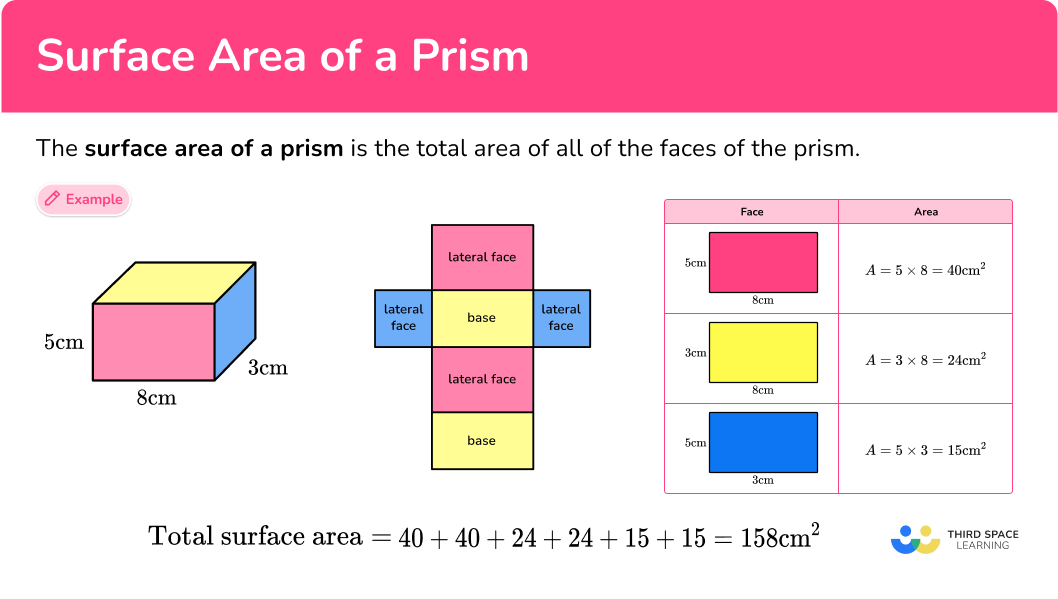## Common Core State Standards

How does this relate to 6 th grade math?

• Grade 6 – Geometry (6.G.A.4)
Represent three-dimensional figures using nets made up of rectangles and triangles, and use the nets to find the surface area of these figures. Apply these techniques in the context of solving real-world and mathematical problems.

## How to calculate the surface area of a prism

In order to work out the surface area of a prism:

1. Calculate the area of each face.
2. Add the area of each face together.
3. Include the units.

## Surface area of a prism examples

### Example 1: surface area of a triangular prism with a right triangle

Calculate the surface area of the triangular prism.

1. Calculate the area of each face.

The area of the front of the prism is \cfrac{1}{2} \, \times 4 \times 3= 6 \mathrm{~cm}^{2}.

The back face is the same as the front face so the area of the back face is also 6 \mathrm{~cm}^{2}.

The area of the bottom is 4 \times 2= 8 \mathrm{~cm}^2.

The area of the left side is 2 \times 3=6 \mathrm{~cm}^2.

The area of the top side is 2 \times 5=10 \mathrm{~cm}^2.

It will make our working clearer if we use a table:

FaceArea
Front \cfrac{1}{2} \times 4 \times 3=6
Back 6
Bottom 4 \times 2=8
Left side 2 \times 3=6
Top 2 \times 5=10

2Add the area of each face together.

Total surface area: 6+6+8+6+10= 36

3Include the units.

The measurements on this prism are in cm , so the total surface area of the prism is 36 \mathrm{~cm}^2 .

### Example 2: surface area of a rectangular prism

Calculate the surface area of the rectangular prism.

A rectangular prism has 6 faces, with 3 pairs of identical faces.

Total surface area: 14+14+21+21+6+6= 82

The measurements on this prism are in m , so the total surface area of the prism is 82 \mathrm{~m}^2 .

### Example 3: surface area of a triangular prism with an equilateral triangle – using a net

Calculate the surface area of the triangular prism. The base of the prism is an equilateral triangle with a perimeter of 16.5 \mathrm{~ft} .

First, use the perimeter of the base to find the length of each side. Since an equilateral triangle has all equal sides, s , the perimeter is s+s+s= 16.5 .

s= 5.5 \mathrm{~ft}

You can unfold the triangular prism, and use the net to find the area of each face:

Remember that the edges in a prism are always equal, so if you were to fold up the net, the 5.5 \mathrm{~ft} side of the triangle would combine to form an edge with each corresponding rectangle – making their lengths equal.

The area of each triangular base: \cfrac{1}{2} \times 4.8 \times 5.5= 13.2

The area of each rectangular lateral face: 10 \times 5.5= 55

If you have trouble keeping track of all the calculations, use a net:

The area of the base is always equal to the opposite base, in this case the triangles. Notice, since the triangle is equilateral, all the rectangular faces are equal as well.

Total surface area: 13.2+13.2+55+55+55= 191.4

The measurements on this prism are in ft , so the total surface area of the prism is 191.4 \mathrm{~ft}^2 .

### Example 4: surface area of a rectangular prism – using a net

Calculate the lateral surface area of the rectangular prism. The base of the prism is a square and one side of the base measures 3 \cfrac{2}{3} \text { inches} .

You can unfold the rectangular prism, and use the net to find the area of each face:

Remember that the edges in a prism are always equal, so if you were to fold up the net, the 3 \cfrac{2}{3} \mathrm{~ft} side of the square would combine to form an edge with each corresponding rectangle – making their lengths equal.

The area of each rectangular lateral face:

\begin{aligned} & 9 \cfrac{4}{5} \times 3 \cfrac{2}{3} \\\\ & = \cfrac{49}{5} \times \cfrac{11}{3} \\\\ & = \cfrac{539}{15} \\\\ & = 35 \cfrac{14}{15} \end{aligned}

Remember, you are only finding the area of the lateral faces, so you do not need to calculate the area of the bases.

If you have trouble keeping track of all the calculations, use a net:

Notice, since the square has all equal sides, all the rectangular faces are equal as well.

Total lateral surface area:

\begin{aligned} & 35 \cfrac{14}{15}+35 \cfrac{14}{15}+35 \cfrac{14}{15}+35 \cfrac{14}{15} \\\\ & =140 \cfrac{56}{15} \\\\ & =143 \cfrac{11}{15} \end{aligned}

The measurements on this prism are in inches, so the total lateral surface area of the prism is 143 \cfrac{11}{15} \text { inches }^2 .

### Example 5: surface area of a parallelogram prism with different units

Calculate the surface area of the parallelogram prism.

A parallelogram prism has 6 faces and, like a rectangular prism, it has 3 pairs of identical faces. The base is a parallelogram and all of the lateral faces are rectangular.

In this example, some of the measurements are in cm and some are in m . You must convert the units so that they are the same. Convert all the units to meters ( m ): 40 \mathrm{~cm}= 0.4 \mathrm{~m} and 50 \mathrm{~cm}= 0.5 \mathrm{~m}.

Total surface area: 0.48+0.48+1.8+1.8+0.75+0.75= 6.06

The measurements that we have used are in m , so the surface area of the prism is 6.06 \mathrm{~m}^2 .

### Example 6: surface area word problem – missing height

Ginny painted an area of 264 \mathrm{~ft}^2 on the rectangular building shown above. She painted all sides, except the bottom. How many feet long is the building

You can unfold the rectangular prism, and use the net to find the area of each face:

The base is a rectangle that measures 6 \mathrm{~ft} by 7 \mathrm{~ft} . The area of each base is 42 \mathrm{~ft}^2 .

Total area of the bases: 42+42=84

Subtract the area of the bases from the total amount of paint Ginny used, to see how much was used on the lateral faces: 264-84=180

The total area of the faces left is 180 \mathrm{~ft}^2 .

Remember that the edges in a prism are always equal, so if you were to fold up the net, the 7 \mathrm{~ft} and 6 \mathrm{~ft} sides of the rectangle would combine to form an edge with each corresponding rectangle – making their lengths equal.

Labeling the missing length as x and show area of 3 lateral faces as expressions:

• 6 \times x or 6 x
• 7 \times x or 7 x
• 7 \times x or 7 x

Together the 3 faces equal 180 \mathrm{~ft}^2 , so the total of the missing areas can be written as:

6x + 7x + 7x= 180

or

20x= 180

Since 20 \times 9= 180 , the missing side length is 9 .

The missing measurement is not the area, it is a side length, which is measured in singular units.

The missing side length is 9 \mathrm{~ft} .

### Teaching tips for the surface area of a prism

• Make sure that students have had time to work with physical 3D models and nets before asking them to solve with surface area.

• Choose worksheets that offer a variety of question types – a mixture of the prism shown or the net, a mixture of solving for the missing surface area or height/length/width, and some word problems.

### Easy mistakes to make

• Calculating volume instead of surface area
Volume and surface area are different things – volume tells us the space within the shape whereas surface area is the total area of the faces. Particularly with rectangular prisms, it is easy to confuse these two topics if a student does not have a complete understanding of the difference.
For example,

Surface Area: Volume:
Left/right side: 4 \times 5=20 \mathrm{~ft}^2 17 \times 4 \times 5=340 \mathrm{~ft}^3
Front/back: 17 \times 5=85 \mathrm{~ft}^2
Top/bottom: 17 \times 4=68 \mathrm{~ft}^2
20+20+85+85+68+68=346 \mathrm{~ft}^2

• Confusing the base and the height of the prism
No matter how the prism is oriented, the height of a prism is always the side length of the rectangular lateral face that does not correspond with the base. The base is always the polygon in which the prism is named after.
For example,
This is a trapezoidal prism. It has a trapezoid as its base. Even though the trapezoids are shown in the front and back position in this image, the trapezoid is still the base and the rectangular sides are the lateral faces.

• Calculating with different units
You need to make sure all measurements are in the same units before calculating surface area.
For example,
Before calculating the surface area, all the units need to be the same. Either the cm needs to be converted to mm or the mm converted to cm.

• Confusing lateral area with total surface area
Lateral area is the area of each of the sides and total surface area is the area of the bases plus the area of the sides. When asked to find lateral area, be sure to only add up the area of the sides – which are always rectangles in a prism.

### Practice surface area of a prism questions

1. Calculate the surface area of the triangular prism: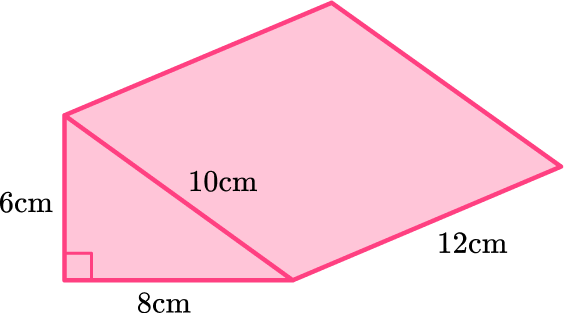288 \mathrm{~cm}^2336 \mathrm{~cm}^2384 \mathrm{~cm}^2408 \mathrm{~cm}^2Calculate the area of each face.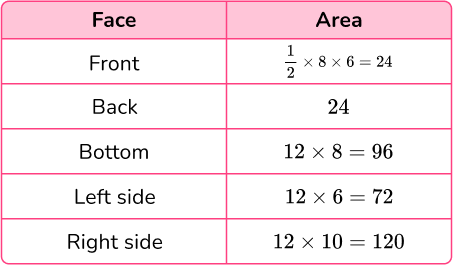Total surface area = 24+24+96+72+120= 336 \mathrm{~cm}^{2}

2. Calculate the surface area of the rectangular prism: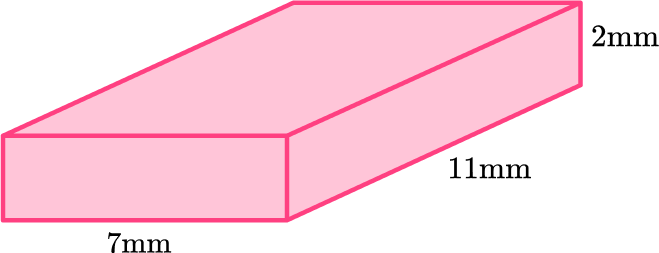154 \mathrm{~mm}^2113 \mathrm{~mm}^2336 \mathrm{~mm}^2226 \mathrm{~mm}^2Calculate the area of each face.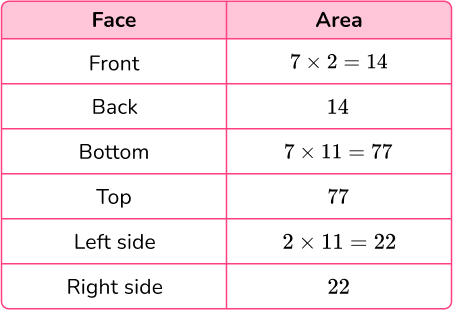Total surface area = 14+14+77+77+22+22= 226 \mathrm{~mm}^{2}

3. Calculate the surface area of the triangular prism: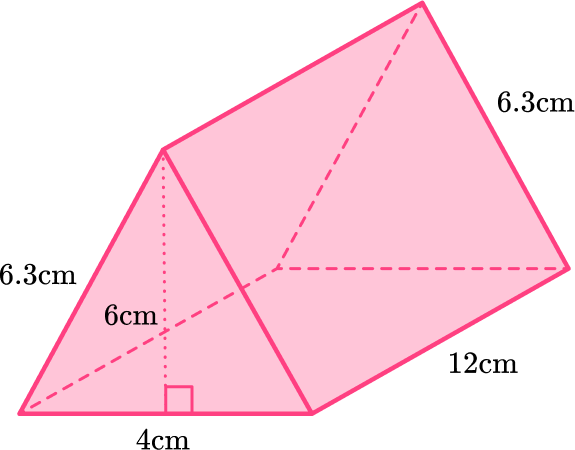168 \mathrm{~cm}^2250.8 \mathrm{~cm}^2223.2 \mathrm{~cm}^2247.2 \mathrm{~cm}^2You can unfold the triangular prism, and use the net to find the area of each face.

Remember that the edges in a prism always fold up together to form the prism – making their lengths equal.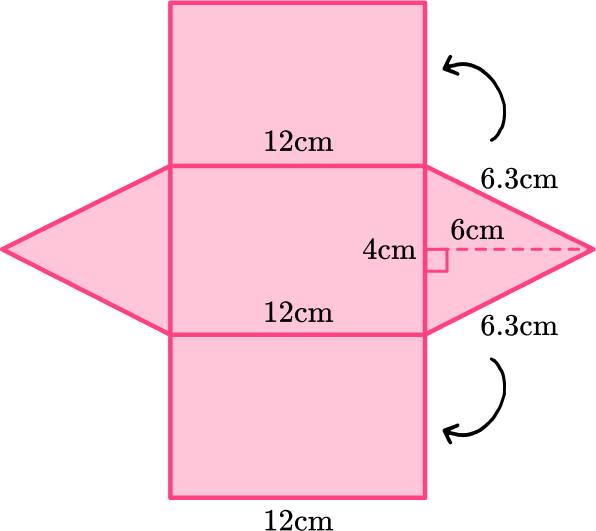The area of each triangular base:

\cfrac{1}{2} \times 4 \times 6= 12

The area of each rectangular lateral face:

12 \times 6.3= 75.6

12 \times 4= 48

12 \times 6.3= 75.6

If you have trouble keeping track of all the calculations, use a net: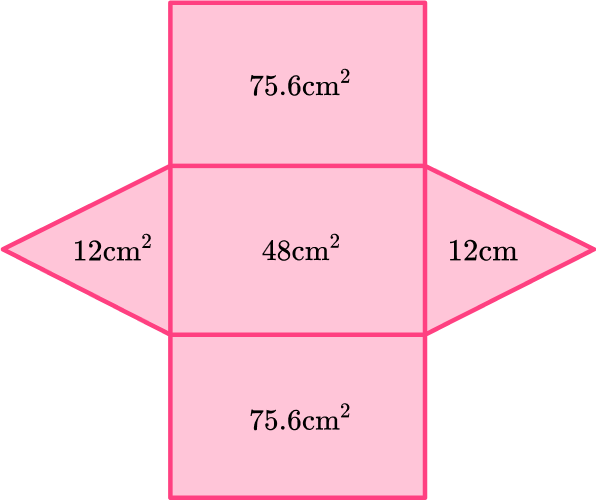Total surface area: 12+12+75.6+48+75.6= 223.2 \mathrm{~cm}^2

4. Calculate the surface area of the rectangular prism: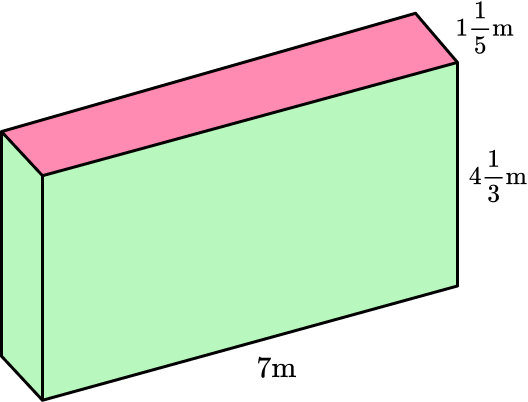87 \, \cfrac{13}{15} \mathrm{~m}^236 \, \cfrac{6}{15} \; m^2138 \, \cfrac{2}{15} \mathrm{~m}^242 \, \cfrac{14}{15} \mathrm{~m}^2You can unfold the rectangular prism, and use the net to find the area of each face.

Remember that the edges in a prism always fold up together to form the prism – making their lengths equal.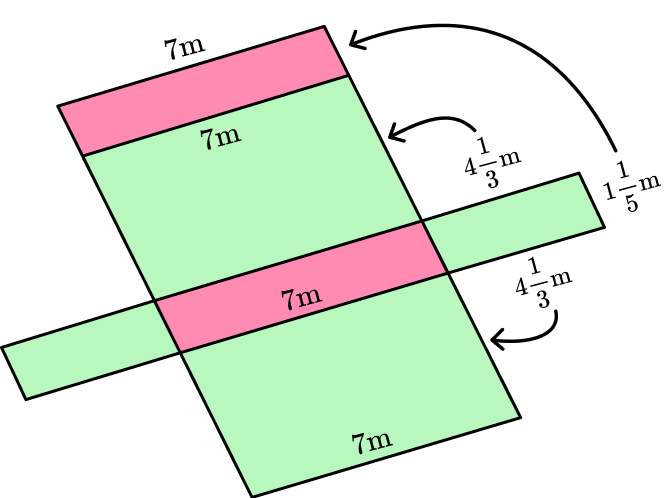The area of each rectangular base:

\begin{aligned} & 1 \cfrac{1}{5} \times 7 \\\\ & =\cfrac{6}{5} \times \cfrac{7}{1} \\\\ & =\cfrac{42}{5} \\\\ & =8 \cfrac{2}{5} \end{aligned}

The area of each rectangular lateral face:

\begin{aligned} & 4 \cfrac{1}{3} \times 7 \\\\ & =\cfrac{13}{3} \times \cfrac{7}{1} \\\\ & =\cfrac{91}{3} \\\\ & =30 \cfrac{1}{3} \end{aligned}

\begin{aligned} & 4 \cfrac{1}{3} \times 1 \cfrac{1}{5} \\\\ & =\cfrac{13}{3} \times \cfrac{6}{5} \\\\ & =\cfrac{78}{15} \\\\ & =5 \cfrac{3}{15} \end{aligned}

If you have trouble keeping track of all the calculations, use a net: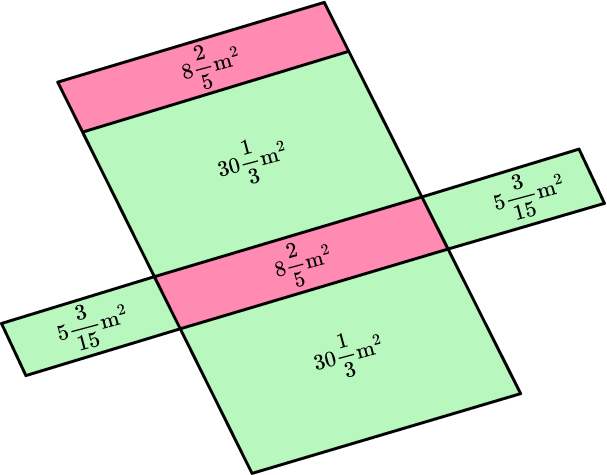Total surface area:

\begin{aligned} & 8 \cfrac{2}{5}+8 \cfrac{2}{5}+30 \cfrac{1}{3}+30 \cfrac{1}{3}+5 \cfrac{3}{15}+5 \cfrac{3}{15} \\\\ & =8 \cfrac{6}{15}+8 \cfrac{6}{15}+30 \cfrac{5}{15}+30 \cfrac{5}{15}+5 \cfrac{3}{15}+5 \cfrac{3}{15}\\\\ & =86 \cfrac{28}{15} \\\\ & =87 \cfrac{13}{15} \mathrm{~m}^2 \end{aligned}

5. Calculate the surface area of the prism: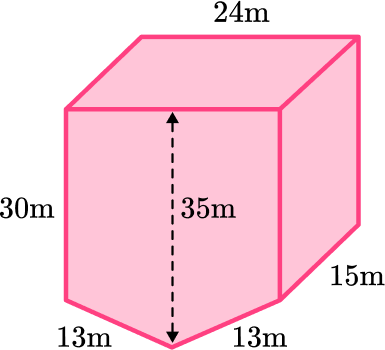3,570 \mathrm{~m}^23,210 \mathrm{~m}^211,700 \mathrm{~m}^23,330 \mathrm{~m}^2Break the base up into a rectangle and a triangle and find the area of each: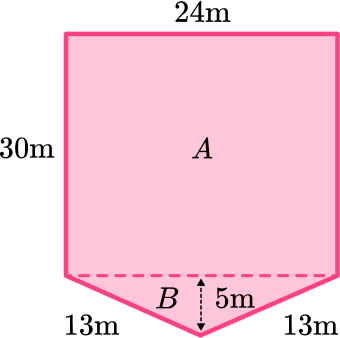Area A :

24 \times 30= 720

Area B :

\cfrac{1}{2} \times 24 \times 5= 60

Total area of the base: 720+60= 780

Calculate the area of each face: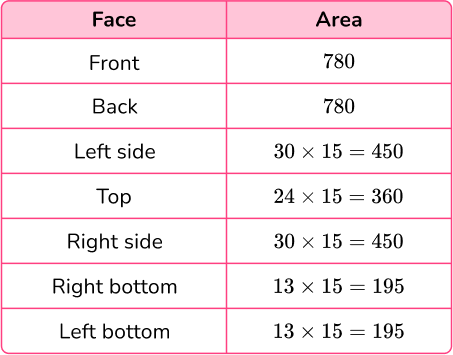Total surface area : 780+780+450+360+450+195+195= 3,210 \mathrm{~m}^{2}

6. Zahir was painting the pentagonal prism below. It took 820 \text { inches}^2 to cover the entire shape. If the area of the base is 140 \text { inches}^2 and each side of the pentagon is 9 \text { inches} , what is the height of the prism?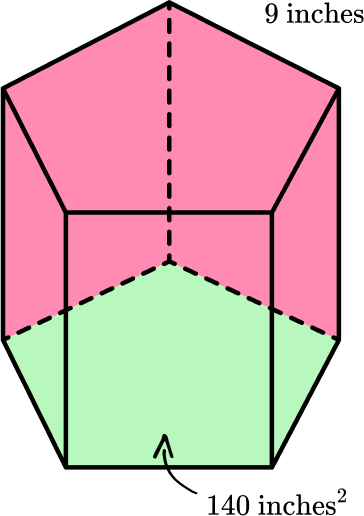120 \text { inches }440 \text { inches }9 \text { inches }12 \text { inches }You can unfold the pentagonal prism, and use the net to find the area of each face: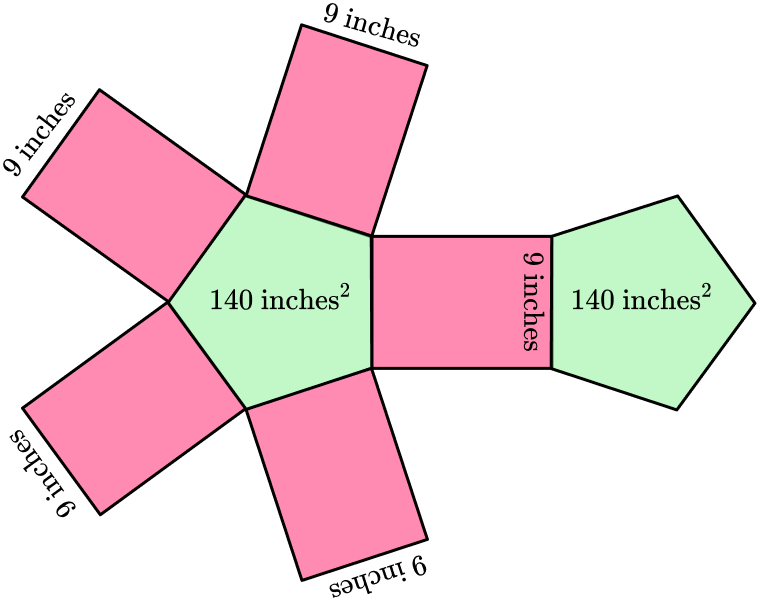Total area of the bases: 140+140= 280

Subtract the area of the bases from the total amount of paint Zahir used, to see how much was used on the lateral faces: 820-280= 540

The total area of the faces left is 540 \text { inches}^2.

Since the 5 faces are congruent, the total for each face can be found by dividing by 5 :

540 \div 5= 108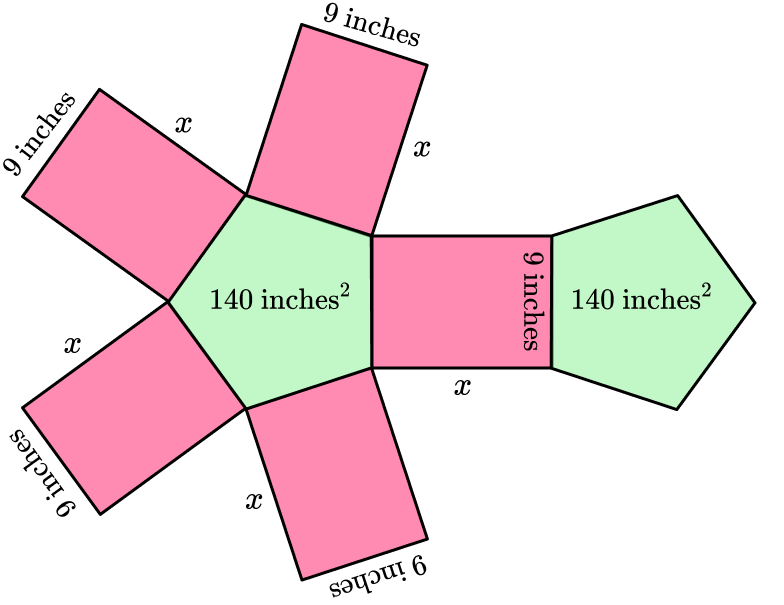Labeling the missing length as x , means the area of each face can be written as 9 \times x or 9 x.

Since each face has an area of 108 \text { inches }^2 , the missing height can be found with the equation: 9 x=108.

Since 9 \times 12=108 , the missing height is 12 inches.

## Surface area of a prism FAQs

Is there a surface area of a triangular prism formula?

The general formula is (S1+ S+ S3) L + bh , but it is not common for students to memorize this formula. It is typically easier for students to remember to find the area of the bases and the three rectangular faces, since these dimensions often vary. Finally, add the area of the faces all together to find the total surface area.

What are some different types of prisms not shown on this page?

There are trapezoidal prisms, which have a trapezoid base. There are also hexagonal prisms, which have a hexagon as a base.

## Still stuck?

At Third Space Learning, we specialize in helping teachers and school leaders to provide personalized math support for more of their students through high-quality, online one-on-one math tutoring delivered by subject experts.

Each week, our tutors support thousands of students who are at risk of not meeting their grade-level expectations, and help accelerate their progress and boost their confidence.